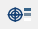# 计算线性化应力

1. 项目树中，打开分析工作台
2. 点击（拾取信息）。
3. 在对话框中，选择线性化应力选项卡。

4. 可选： 输入膜应力、膜+弯曲应力和峰值应力的失效准则，以查看创建的段的通过/失败状态。默认值是使用材料的屈服应力计算的。

膜应力 = 屈服应力的 2/3

膜 + 弯曲应力 = 屈服应力

峰值应力 = 2 倍屈服应力

当模型中存在多种材料时，将使用屈服应力最高的材料来计算默认允许量。

5. 在“应力线性化段”下，使用以下任意一种方式定义线段：
选项 步骤
垂直于表面 激活垂直于表面单选按钮。

SimSolid 会发射一条垂直于曲面并穿过零件的光线。

自定义
1. 激活自定义单选按钮。
2. 模型视窗中，拾取零件上的入口点和出口点。

在对话框中填充入口点和出口点坐标。

垂直于基准点
1. 激活垂直于基准点单选按钮。
2. 在下拉菜单中，选择一个点集。
线性化的局部应力是动态计算的，并发布在对话框中，用于定义的每个线段。默认情况下，不变量显示在表格中。
6. 选中显示线性化的应力张量分量复选框可查看应力张量分量和不变量。
提示： 直线状态会根据失效准则显示通过或失败。失效准则仅根据不变量进行评估。
7. 可选： 将线性化应力信息保存为 CSV 文件。
1. 点击保存为 CSV
2. 在对话框中，输入文件名，然后选择保存位置。
3. 点击保存

## 计算方法

SimSolid 线性化应力的计算方法。

1. 应力是通过在局部坐标系中沿直线的所有点上的插值来提取的。局部坐标系以应力线性化段的起始点和结束点为基础。
系统的 X 轴沿着从入口点到出口点的段。其他两个轴的计算方法如下：
• 如果局部 x 轴不平行于全局 y 轴：

Zlocal = Xlocal x Yglobal

Ylocal = Zlocal x Xlocal

• 如果局部 x 轴平行于全局 y 轴：

如果局部 x 沿着正的全局-y，则局部 y 轴 (Ylocal) 是负的全局-x，反之亦然。

Zlocal = Xlocal x Ylocal

2. 根据上述提取的应力值，采用数值积分的方法计算出入口点和出口点的平均膜应力张量 + 弯曲应力张量。

${\sigma }_{i}^{m}\text{\hspace{0.17em}}=\text{\hspace{0.17em}}\frac{1}{L}{\int }_{-L/2}^{L/2}{\sigma }_{i}dx$

${\sigma }_{iS}^{b}\text{\hspace{0.17em}}=\text{\hspace{0.17em}}-\frac{6}{{L}^{2}}{\int }_{-\frac{L}{2}}^{\frac{L}{2}}{\sigma }_{i}xdx$

${\sigma }_{iE}^{b}\text{\hspace{0.17em}}=\text{\hspace{0.17em}}-{\sigma }_{iS}^{b}$

${\sigma }_{i}^{m}$ = 膜应力的第 i 个分量

${\sigma }_{i}$ = 提取应力值的第 i 个分量

${\sigma }_{iS}^{b}$ = 入口弯曲应力的第 i 个分量

${\sigma }_{iE}^{b}$ = 出口弯曲应力的第 i 个分量

L = 应力线性化段的长度

X = 某一点沿段的位置

3. 还计算了入口和出口处的峰值应力、膜应力和弯曲应力。

${\sigma }_{iS}^{p}\text{\hspace{0.17em}}=\text{\hspace{0.17em}}{\sigma }_{iS}\text{\hspace{0.17em}}-\text{\hspace{0.17em}}\left({\sigma }_{i}^{m}\text{\hspace{0.17em}}+\text{\hspace{0.17em}}{\sigma }_{iS}^{b}\right)$

${\sigma }_{iE}^{p}\text{\hspace{0.17em}}=\text{\hspace{0.17em}}{\sigma }_{iE}\text{\hspace{0.17em}}-\text{\hspace{0.17em}}\left({\sigma }_{i}^{m}\text{\hspace{0.17em}}+\text{\hspace{0.17em}}{\sigma }_{iE}^{b}\right)$

${\sigma }_{iS}^{p}$ = 入口峰值应力的第 i 个分量

${\sigma }_{iE}^{p}$ = 出口峰值应力的第 i 个分量

4. 最后，计算了膜应力、膜 + 弯曲应力和峰值应力的不变量。